Next: Numerical instabilities Up: Numerical treatment of the Previous: The problem inside a

## The Numerov algorithm for the HFB equation

The Numerov (Cowell) method  is the standard technique for a precise integration of second-order differential equations; here we only give some details pertaining to its application to the system of two HFB equations (34). Let function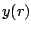be the solution of the differential equation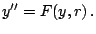(59)

The Numerov algorithm is based on the finite difference formula for three consecutive points on a mesh with step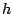,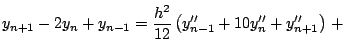o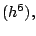(60)

where o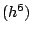means that the error in this relation is of the order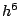. Combining this formula with (60), one obtains the relation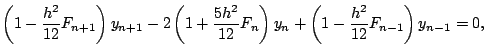(61)

which is obviously also valid if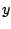is a vector and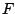a square matrix. Introducing notations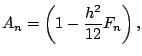(62)

and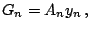(63)

one obtains the recurrence relation (Numerov iterations),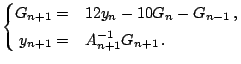(64)

In the present applications,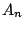is a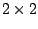matrix (position and energy dependent) and the calculation of its inverse could be the bottleneck of the procedure if it is not computed cleverly. This can be done in the following way. Using the notations introduced in Sec. 2.5, one can write matrixin an explicit form as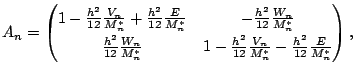(65)

whereby its inverse is given by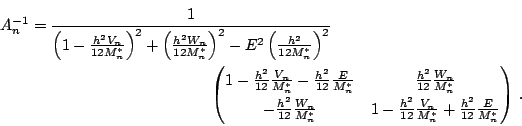It appears clearly that a lot of time can be saved by calculating and storing the energy independent terms,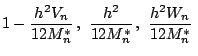and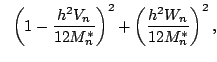(66)

only once in each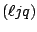-block; then the energy-dependent terms can be in each iteration calculated very rapidly.

The eigen energies are found by integrating two linearly independent solutions,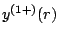and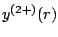, from the origin to a given point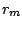, called the matching point. These two solutions are selected by imposing that either the first or the second component of the quasiparticle wave function vanishes near the origin, i.e.,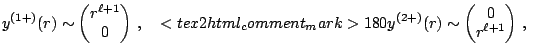for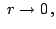(67)

whereby we have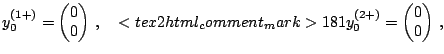(68)

and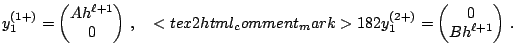(69)

Next, another two linearly independent solutions,and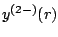, are found by the backward integration from the wall of the box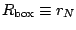to, again imposing that either the first or the second component of the quasiparticle wave function vanishes near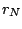for the Dirichlet boundary conditions, i.e.,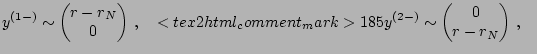for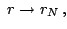(70)

whereby we have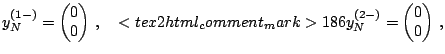(71)

and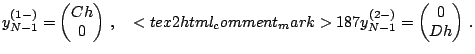(72)

For the Neumann boundary conditions, at the left-hand-sides of Eqs. (71)-(73) one replaces functions by derivatives. Finally, one obtains four solutions valid everywhere except at the matching pointand depending on the four constants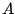,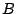,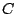, and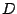.

The boundary conditions (69)-(70) and (72)-(73) determine the initial values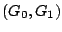and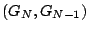, respectively, needed to start the Numerov iterations of (65). Obviously, a correct limit should be taken when calculating values of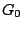from Eq. (64). It turns out that one obtains=0 for all partial waves except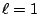, which is the case that deserves a little more attention. For example, focusing our attention on one of the solutions,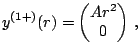(73)

we use the fact that in the vicinity of the origin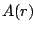is dominated by the centrifugal term, so that its diagonal matrix elements are close to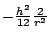. This leads to the result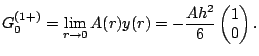(74)

An analogous result can be obtained for the other solution at the origin.

The eigenenergies are found by requiring that the full solution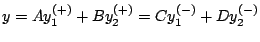and its first derivative are continuous at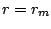(see for example ). This matching conditions read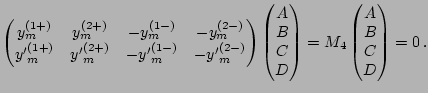(75)

The HFB quasiparticle wave functions having two components, the matching conditions amount to searching for zeros of the determinant of the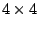matrix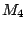in function of the quasiparticle energy. For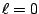, the matching pointis chosen to be near the point where the central part of the Hartree-Fock potential is half of its maximum, while for increasing values of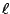it is gradually shifted to the exterior. A systematic search allows to find the intervals where the zeros of this determinant are located, and then the Newton method is used to find their exact locations to an arbitrary precision.

For vanishing determinant, Eq. (76) is used to determine three of the multiplicative constants in terms of the fourth one. This is done by extracting a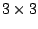matrix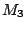out ofand inverting it. Among the four possible choices one may have forwe retain the one for which the product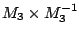is closest to unity. Finally, the fourth multiplicative constant is fixed by the normalization condition of the wave function.

When approaching the self-consistent solution, the eigenenergies do not change very much between two consecutive Bogolyubov iterations. We take advantage of this fact by keeping in memory the intervals where the solutions are located and using them as initial guesses for the next iteration. This results in finding solutions for eigenenergies faster and faster as we get closer to the converged self-consistent state.Next: Numerical instabilities Up: Numerical treatment of the Previous: The problem inside a
Jacek Dobaczewski 2005-01-23